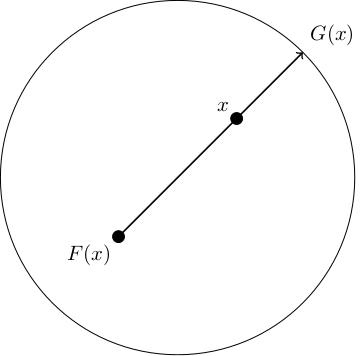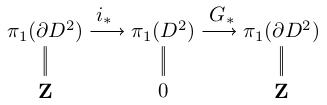# 1.08 Brouwer's fixed point theorem

Below the video you will find accompanying notes and some pre-class questions.

# Notes

## Brouwer's fixed point theorem

(0.30) Let $$F\colon D^2\to D^2$$ be a continuous map, where $$D^2=\{(x,y)\in\mathbf{R}^2\ :\ x^2+y^2\leq 1\}$$ is the 2-dimensional disc. Then there exists a point $$x\in D^2$$ such that $$F(x)=x$$ (a fixed point).
(1.40) Assume, for a contradiction, that $$F(x)\neq x$$ for all $$x\in D^2$$. Then we can define a map $$G\colon D^2\to\partial D^2$$ (where $$\partial D^2$$ denotes the boundary circle) as follows: consider the ray starting at $$F(x)$$, passing through $$x$$ and let $$G(x)$$ to be the unique point of intersection of this ray with $$\partial D^2$$.(3.45) If there were a fixed point, we would not know which ray to draw (as $$F(x)=x$$), so this map is only well-defined because we have assumed there are no fixed points.

(3.58) We claim that:

1. $$G$$ is continuous; this is intuitively clear, but requires proof (below).
2. $$G(x)=x$$ if $$x\in\partial D^2$$; this is clear, because if $$x\in\partial D^2$$, no matter where $$F(x)$$ is, the ray from $$F(x)$$ to $$x$$ intersects the boundary at $$x$$, so $$G(x)=x$$.
(5.25) Given these two claims, we get a contradiction as follows. Let $$i\colon\partial D^2\to D^2$$ be the inclusion map of the boundary; the composition $$G\circ i$$ equals the identity map on $$\partial D^2$$ by claim 2. This implies $id=G_*\circ i_*\colon\pi_1(\partial D^2)\to\pi_1(\partial D^2).$ We have $$\pi_1(\partial D^2)=\mathbf{Z}$$, and this is saying that the identity map on the integers factors through the map $$i_*\colon\pi_1(\partial D^2)\to\pi_1(D^2)=0$$:(7.30) This implies that $$G_*\circ i_*=0$$. This is impossible, as it implies $$n=G_*(i_*(n))=G_*(0)=0$$ for all $$n\in\mathbf{Z}$$. Therefore we have a contradiction, and we have proved Brouwer's fixed point theorem, modulo claim 1.

(9.20) It remains to prove claim 1 (that $$G$$ is continuous). We can write $$G$$ as a composition $$H\circ j$$ where:

• $$j\colon D^2\to D^2\times D^2\setminus\{(x,x)\ :\ x\in D^2\}$$ is the map $$j(x)=(x,F(x))$$.
• (11.30) $$H\colon D^2\times D^2\setminus\{(x,x)\ :\ x\in D^2\}$$ is the map defined as follows. Given two distinct points $$x\neq y$$ in $$D^2$$, let $$H(x,y)$$ denote the point where the ray from $$y$$ through $$x$$ intersects $$\partial D^2$$.
(12.25) By definition, we have $$G(x)=H(x,F(x))=H(j(x))$$. By the properties of the product topology, the map $$j$$ is continuous (more generally, if $$p$$ and $$q$$ are continuous then $$(p,q)$$ is continuous). It therefore suffices to show that $$H$$ is continuous.

(13.25) We can write $$H$$ explicitly in coordinates. The ray from $$y$$ through $$x$$ is given in parametric form by $$y+t(x-y)$$. The condition that the ray meets the boundary of the disc is $|y+t(x-y)|^2=1,$ which is a quadratic equation in $$t$$: $t^2|x-y|^2+2t(x-y)\cdot y+|y|^2-1=0.$ (Note that the leading term is never zero since $$x\neq y$$). This has solution $t_\pm=\frac{-2(x-y)\cdot y\pm\sqrt{4((x-y)\cdot y)^2-4|x-y|^2(|y|^2-1)}}{2|x-y|^2}$ We are only interested in solutions with $$t\geq 0$$ as the ray only points in one direction. Therefore the point $$H(x,y)$$ is $$y+t_+(x-y)$$, which is now expressed purely in terms of rational functions and surds, all of which are continuous.

# Pre-class questions

1. Brouwer's fixed point theorem tells us that continuous maps between 2-discs have fixed points. Is the same true for maps between 2-dimensional annuli? (An annulus is $$S^1\times[0,1]$$).
2. Brouwer's fixed point theorem also holds for maps $$F\colon D^n\to D^n$$ where $$D^n$$ is the $$n$$-dimensional disc; can the proof above be adapted to cover this case, or are new ideas required?